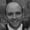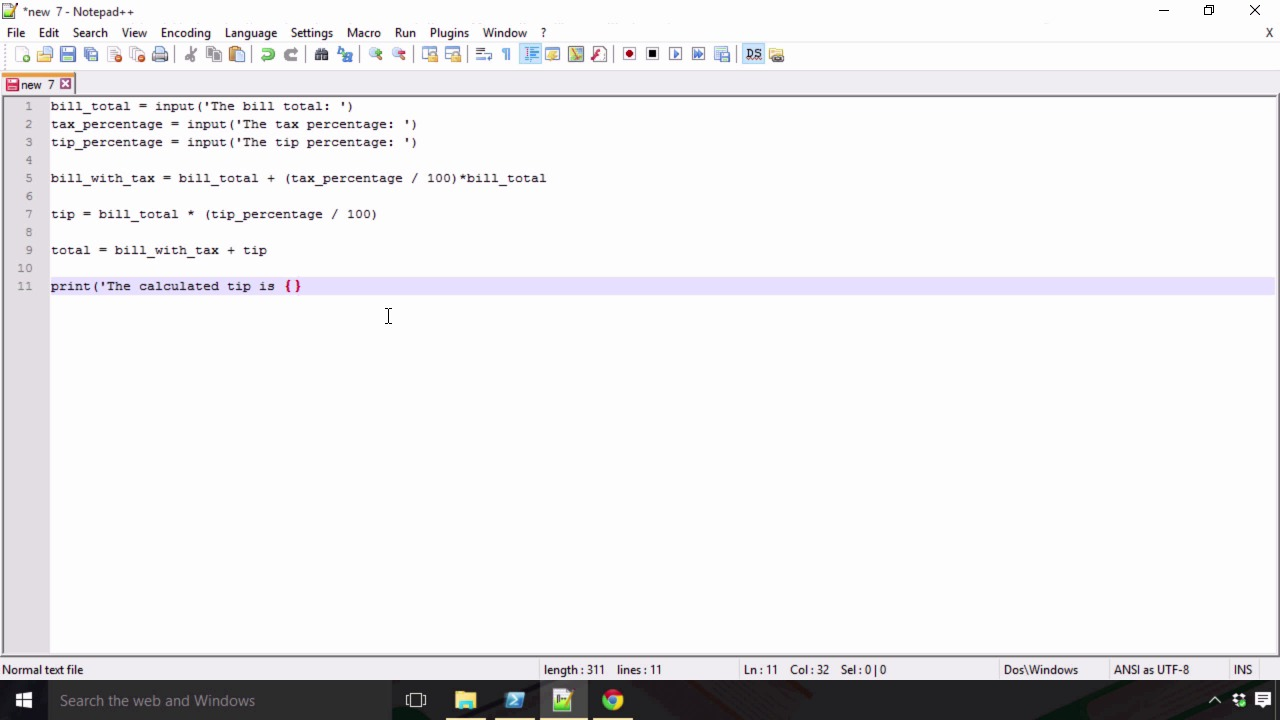FREELessons: 48Length: 7.6 hours• Overview
• Transcript

# 2.6 Building a Tip Calculator

With the knowledge you've already acquired in this chapter, you already could write hundreds of useful applications. We don't have time to do so many in this lesson, so we'll have to settle for just one: a simple tip calculator.

## 9.Connecting to Network Services3 lessons, 34:27

### 9.2Getting Websites With HTTP12:57

At this point in the course we now have all the tools necessary to be able to build a very simple application that we can use, and that we can share with others to use. And ultimately at the end of the day, that's why we write software, that's why we do what we do to solve problems to make life easier for not only ourselves, but also other people. So now I have kind of started to play around with the concept of a calculator application that we could use if we, say, went out on the town with some friends, we went to a restaurant, had some food, had some drinks, did whatever, and then ultimately at the end of the night had to settle up and pay a bill. Now at the end of a long night, I can be rather tired and have a difficult time doing mathematics in my head. Sure, I could go down and punch it all into my phone and maybe that would make a lot more sense then lugging around something that I could run my Python script on but you'll just have to bear with me. So this is how we're going to do it, we're going to structure this in such a way where we're going to build an application that's going to take in the total bill amount for the evening, as well as the tax percentage. And then the percentage of a tip that we might want to leave for the waiter or waitress at the end of the evening. So, this should be a fairly interesting exercise. So the first thing that we need to do is we need to get some input from the users. So the first thing we wanna get is the bill. So, we wanna get the bill total and we're gonna specify that as a variable and we're going to use our input function again. And that its going to say the bill total just like that and then we're going to say the tax underscore percentage is going to be equal to input the tax percentage like that and you know just to be a little bit more complete in our application we're going to specify this as being the bill total. Then we're also going to ask for a tip percentage just to allow us to be able to include the value of the tip should we want to and we'll say the tip percentage. Now you can get very fancy and do a lot of complicated logic in here. And we haven't really learned enough to this point to be able to add some of that logic in there, so we're just going to make a few assumptions at this point that we're going to pass in valid values for all of these, so we're going to pass in actual integers or floating point numbers. And we're not gonna pass in any sort of numbers, because we haven't really learned other conditional statements and how to handle things like that in validations. So, we're going to make that assumption. We're also going to make the assumption, that we are going to include the tip amount that we calculate in the total for the end of the evening. So having said that let's start to do some calculations. So we're going to say that the bill_with_tax is going to be equal to the bill_score total plus the tax_percentage. And we're going to make the assumption that the end user is going to enter it in in integer and decimal form so 7% would be 7.00 or 5 and a half percent would be 5.50, and that they're gonna give it to us in that sort of format. So if they were to do that, we would have to then divide that value by 100 to get the actual percentage. So 5.50 would turn into 0.055. So then I can multiply that by the bill total. And that's going to give me the bill with tax. So now that I have the bill with tax. I am going to also calculate the tip percentage. And the tip percentage is going to be based on the percentage of the bill total. So we're going to say that the tip is going to be equal to bill total times and once again we're going to take the tip percentage we're gonna divide that by 100 because we're gonna make the same assumption that the tip percentage is being passed in the same way that tax percentage is. So now we have the tip value. So now we can say that the total is going to be equal to bill with tax plus tip. And then we'll just do a little bit of printing down here at the bottom we'll say print. The calculated tip is and then we're gonna use our little curly braces and then we're going to format tip because recall, I really don't want to print out some really long value, I just want to round it to two decimal places. So in this case we're going to be working with the first argument passed into the format function. And we're going to format it to two decimal places and it's going to be a floating point number. And then we are going to print out another line print. The total amount due is, and we'll do the same thing again here. And we're going to use format and this time we're gonna format the total. And then we're going to specify that this is going to be the first argument once again with the zero index, so we're going to round that, or format that to two floating point values. So now we're going to save this, so we'll save this as tip calc. And we're once again going to make sure that this is a Python file, so we'll save them. So now what I want to do is I want to run this, but once again I'm going to warn you that the output may be a little strange. So we're going to say, Python. And we want to run tip calc. So, the bill total. Let's just make this easy. We'll say, is ten dollars. The tax percentage is 10%, that's awfully high but just to make it a little bit interesting and for argument's sake, let's just say that the tip percentage is gonna be 0 for this time. Now I'm gonna hit enter and something here went wrong. Now the nice thing about looking at errors that come from Python is it's usually pretty good about telling you where and how things went wrong. So as you can see here, the error happened on line five where it says bill with tax bill total plus. And then our little math here, unsupported operand types for division string and integer. So that will remind you that when we got the input from the user, these things are strings but we don't want them to be strings, they're being entered as floating point numbers. So I'm just gonna make some a few little changes here, so we're going to put in float here. And we're going to copy this, copy and we'll paste it here. Close off that parenthesis, and we'll do the same thing here and we'll save that. Once again we'll do the same for bill_total here and here. And on the tip percentage, and on the end. And we'll save that. So once we've done those things. Now, bill with tax will be a floating point number, as well, will tip. So let's go ahead and save that, now. So we'll come back into our application. Let's clear this out, let's go ahead and run it again. So we'll say bill with total was $10, the tax percentage is 10%, and the tip percentage is going to be zero. So now the calculated tip is zero which is what we expected, and the total amount due is$11, which means 10% of the bill total would be $1 and if I were to add to that, that$1, then we would get 11. So that seems to make sense. So then one final time we'll run this again just to make sure that the other calculations go well. So let's say the bill total was $10 and the tax percentage is once again 10%, and then the tip percentage we do as ten as well. We're now going to get$12 because the calculated tip is a dollar, 10% of ten dollars is one dollar. And then the same with the tip percentage, 10% of $10 is$1 we add those two \$1 to the total and we get 12. So there you have it, now just by learning some basic information about some of the foundational data types that come built in with Python, in this case namely strings and numbers, and then learning a little bit about the standard input and formatting magic that comes out of the box as well, you're able to build an actual application. Now isn't that easy? Now this is definitely one of the reasons why Python is so endearing. And is very pushed on people early on because it makes learning how to do very simple tasks and learning how to do programming in general relatively easy as it doesn't take very long to go from zero to writing an application. But now that we've gone this far it's a little bit limiting, we need to learn some of the more aspects of the language. Namely how to start working with conditionals and loops and all those types of things to really start to add some logic to our applications and that's exactly where we're going to start covering in the next section.